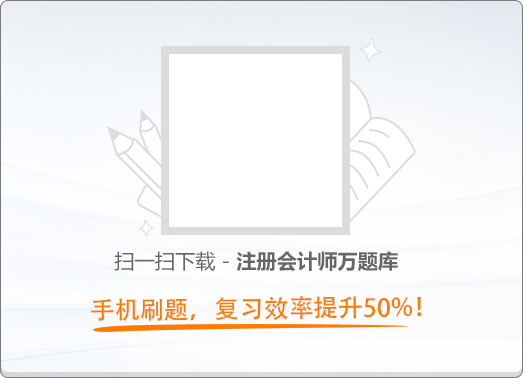120:002010年注册会计师《税法》真题

1
(单项选择题)

• A. 税法是引起法律关系的前提条件，税法可以产生具体的税收法律关系
• B. 税收法律关系中权利主体双方法律地位并不平等，双方的权利义务也不对等
• C. 代表国家行使征税职责的各级国家税务机关是税收法律关系中的权利主体之一
• D. 税收法律关系总体上与其他法律关系一样，都是由权利主体、权利客体两方面构成
• A
• B
• C
• D

2
(单项选择题)

• A.

居民甲应缴纳契税1万元

• B.

居民乙应缴纳契税1万元

• C.

居民甲应缴纳契税10万元

• D.

居民乙应缴纳契税10万元

• A
• B
• C
• D

3
(单项选择题)

• A. 扣缴义务人对纳税人的应扣未扣税款应由扣缴义务人予以补缴
• B. 外籍个人从外商投资企业取得的股息、红利所得应缴纳个人所得税
• C. 在判断个人所得来源地时对不动产转让所得以不动产坐落地为所得来源地
• D. 个人取得兼职收入应并入当月“工资、薪金所得"应税项目计征个人所得税
• A
• B
• C
• D

4
(单项选择题)

• A. 免税法可以分为全额免税法和累进免税法两种
• B. 免税法以承认居民管辖权独占地位为前提，被世界各国普遍采用
• C. 抵免法以承认居民管辖权优先为前提条件，但并不承认其独占地位
• D. 抵免法中的直接抵免是指对跨国纳税人在非居住国非直接缴纳的税款，允许部分冲抵其居住国纳税义务
• A
• B
• C
• D

6
(单项选择题)

• A.

2.8万元

• B.

3.15万元

• C.

4.6万元

• D.

6.09万元

• A
• B
• C
• D

7
(单项选择题)

• A. 出口关税税额
• B. 单独列明的支付给境外的佣金
• C. 货物在我国境内输出地点装载后的运输费用
• D. 货物运至我国境内输出地点装载前的保险费
• A
• B
• C
• D

8
(单项选择题)

• A.

21.2万元

• B.

27.6万元

• C.

29.7万元

• D.

44.4万元

• A
• B
• C
• D

9
(单项选择题)

• A.

216000元

• B.

224000元

• C.

264000元

• D.

328000元

• A
• B
• C
• D

10
(单项选择题)

• A.

20％

• B.

30％

• C.

50％

• D.

100％

• A
• B
• C
• D

11
(单项选择题)

• A. 因税务机关责任，致使纳税人少缴税款的，税务机关在3年内可要求纳税人补缴税款，但不加收滞纳金
• B. 因税务机关责任，致使纳税人少缴税款的，税务机关在3年内可要求纳税人补缴税款并按银行同期利率加收滞纳金
• C. 对于纳税人偷税、抗税和骗取税款的，税务机关在20年内可以追征税款、滞纳金；有特殊情况的，追征期可延长到30年
• D. 因纳税人计算等失误，未缴或者少缴税款的，税务机关在3年内可以追征税款、滞纳金；有特殊情况的，追征期可延长到l0年
• A
• B
• C
• D

12
(单项选择题)

• A. 8000元
• B. 9200元
• C. 13040元
• D. 16640元
• A
• B
• C
• D

13
(单项选择题)

• A.

外汇收支鉴证

• B.

银行储蓄存款鉴证

• C.

企业会计报表鉴证

• D.

企业所得税财产损失鉴证

• A
• B
• C
• D

14
(单项选择题)

• A. 税务咨询报告对委托人的纳税起决定性的作用
• B. 税务咨询报告对委托人的纳税起指导和参考的作用
• C. 税务咨询报告对税务机关的决策起举足轻重的作用
• D. 税务咨询报告对税务机关执法力度的掌握起关键的作用
• A
• B
• C
• D

15
(单项选择题)

• A. 出纳长款收入
• B. 保险追偿款收入
• C. 财产保险费收入
• D. 出口信用保险收入
• A
• B
• C
• D

16
(单项选择题)

• A. 电动汽车
• B. 卡丁车
• C. 高尔夫车
• D. 小轿车
• A
• B
• C
• D

17
(单项选择题)

• A.

开采数量

• B.

实际产量

• C.

计划产量

• D.

销售数量

• A
• B
• C
• D

18
(单项选择题)

• A. 规划申报项目
• B. 审批备案项目
• C. 商业推广项目
• D. 设计建筑项目
• A
• B
• C
• D

19
(单项选择题)

• A. 企业之间支付的管理费
• B. 银行内营业机构之间支付的利息
• C. 企业内营业机构之间支付的租金
• D. 企业内营,机构之间支付的特许权使用费
• A
• B
• C
• D

20
(单项选择题)

• A. 采取银行按揭方式销售开发产品的，其首付款应于实际收到日确认收入实现
• B. 采取支付手续费方式销售开发产品的，以代销方取得销售收入日确认收入实现
• C. 采取视同买断方式委托销售开发产品的，以受托方取得销售收入日确认收入实现
• D. 采取分期收款方式销售开发产品的，付款方早于合同日付款的按合同日确认收入实现
• A
• B
• C
• D